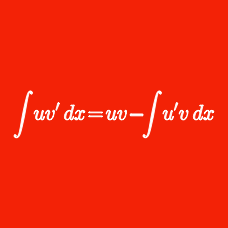Calculus

# Integration with Partial Fractions

If $f(x)=\int \frac{4x}{x^2-2x-3} dx$ and $$f(0)=15 \ln 3,$$ what is the value of $$f(2)?$$

If $$f(x)$$ is a function such that $f'(x)=\frac{1}{25x^2-1}$ and $$f(0)=7,$$ what is the value of $$f(1)?$$

What is the indefinite integral $\int \frac{x+2}{(x-4)(x-5)} dx?$ (Use $$C$$ for the constant of integration.)

If $f(x)=\int \frac{12x^2}{(x-1)(x^2+x+1)} dx$ and $$f(0)=0,$$ what is the value of $$\displaystyle{e^{\frac{f(9)}{4}}}?$$

If $$a$$ is a nonzero real number, what is $\int \frac{35}{x^2 - a^2} dx?$

Details and assumptions

Use $$C$$ as the constant of integration.

×

Problem Loading...

Note Loading...

Set Loading...